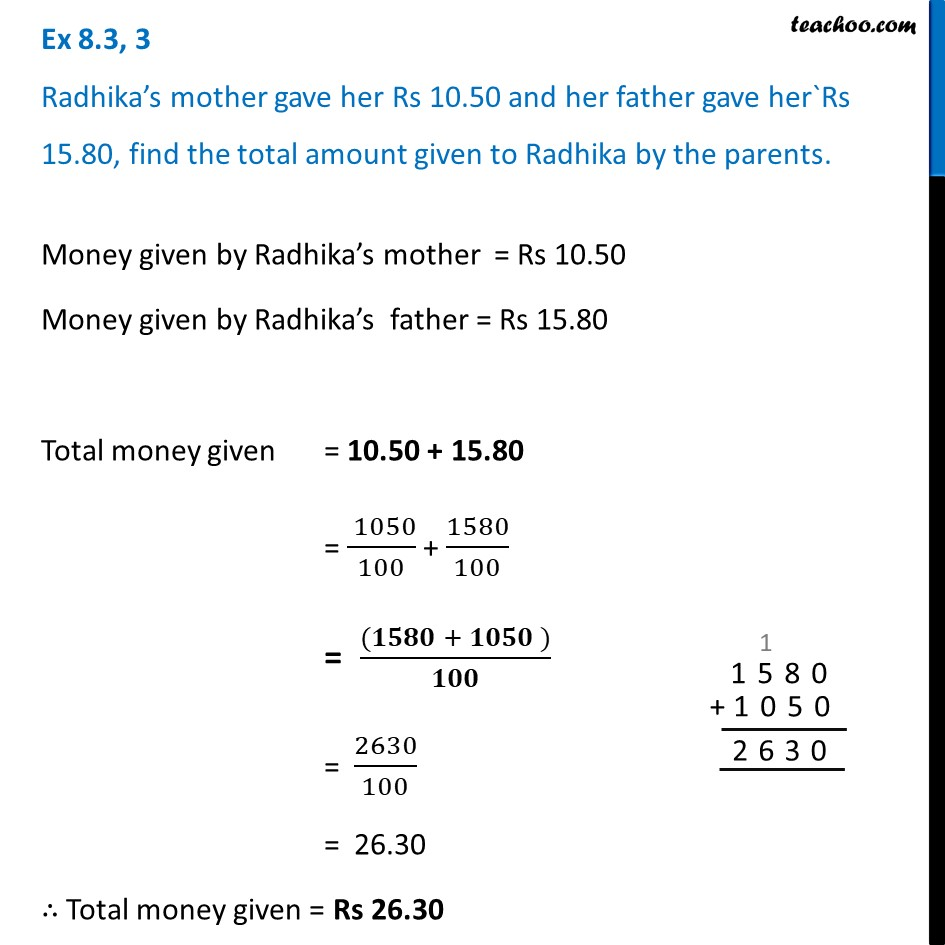Ex 8.3

Chapter 8 Class 6 Decimals
Serial order wiseLearn in your speed, with individual attention - Teachoo Maths 1-on-1 Class

### Transcript

Ex 8.3, 3 Radhika’s mother gave her Rs 10.50 and her father gave her`Rs 15.80, find the total amount given to Radhika by the parents.Money given by Radhika’s mother = Rs 10.50 Money given by Radhika’s father = Rs 15.80 Total money given = 10.50 + 15.80 = ( 1050)/100 + 1580/100 = ((𝟏𝟓𝟖𝟎 + 𝟏𝟎𝟓𝟎 ))/𝟏𝟎𝟎 = 2630/100 = 26.30 ∴ Total money given = Rs 26.30